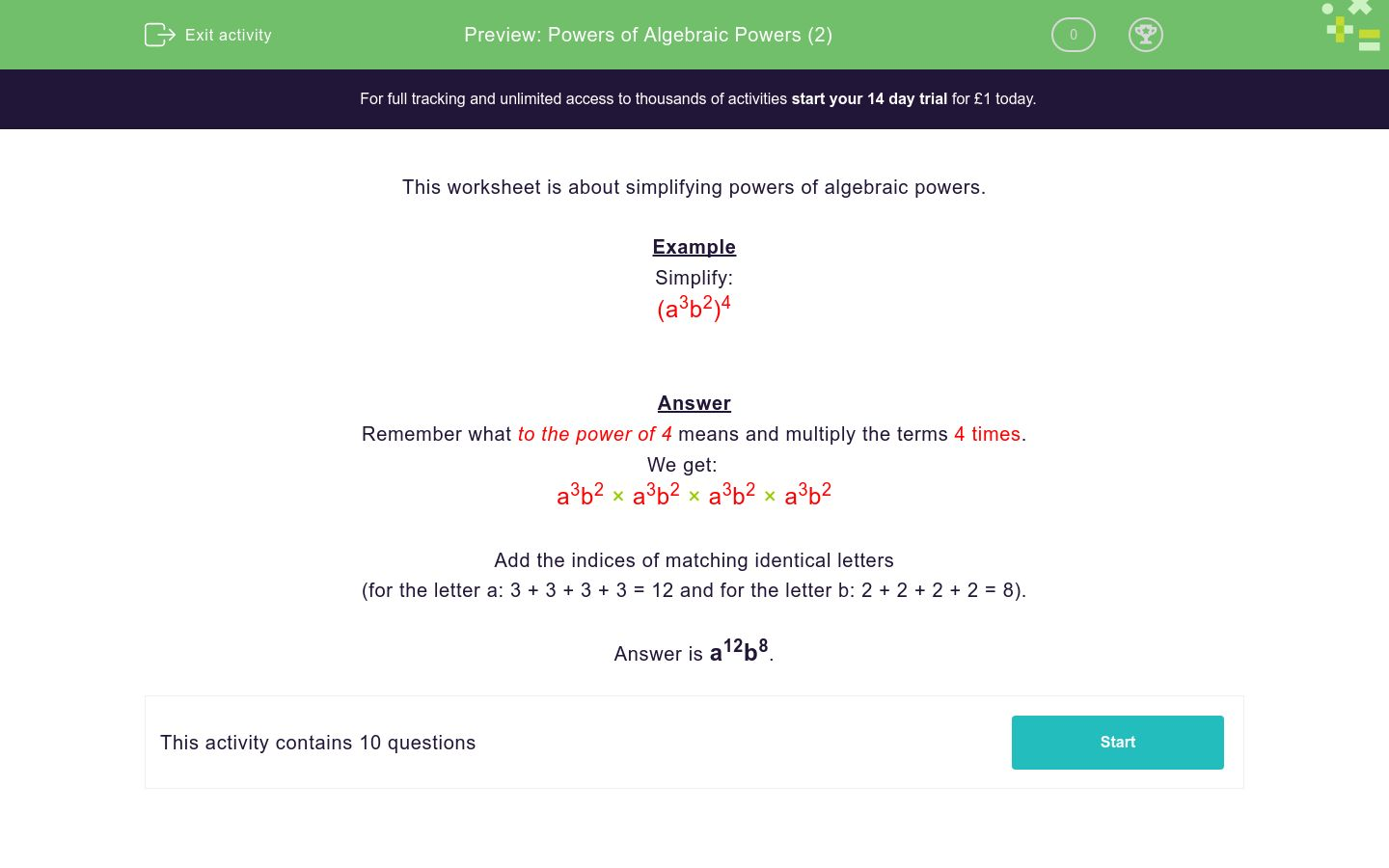# Powers of Algebraic Powers (2)

In this worksheet, students must simplify powers of algebraic powers.Key stage:  KS 3

Curriculum topic:   Algebra

Curriculum subtopic:   Simplify Algebraic Expressions to Maintain Equivalence

Difficulty level:### QUESTION 1 of 10

This worksheet is about simplifying powers of algebraic powers.

Example

Simplify:

(a3b2)4

Remember what to the power of 4 means and multiply the terms 4 times.

We get:

a3b2 × a3b2 × a3b2 × a3b2

Add the indices of matching identical letters

(for the letter a: 3 + 3 + 3 + 3 = 12 and for the letter b: 2 + 2 + 2 + 2 = 8).

Simplify:

(a3b)2

a32b2

a5b

a6b2

Simplify:

(a2b2)2

a22b22

a4b4

a4b2

Simplify:

(a3b2)2

a32b22

a6b4

a6b2

Simplify:

(ab2)2

ab4

a2b4

ab2

Simplify:

(cb2)3

c3b6

c2b4

cb6

Simplify:

(c3d2)3

c3d6

c6d5

c9d6

Simplify:

(f3d2)4

f7d6

f6d5

f12d8

Simplify:

(f3g)4

f7g4

f12g4

f12g

Simplify:

(p3q)6

p18q6

p9q6

p18q

Simplify:

(p3q4)7

p10q11

p21q11

p21q28

• Question 1

Simplify:

(a3b)2

a6b2
EDDIE SAYS
a3b × a3b
a3 × a3 × b × b
• Question 2

Simplify:

(a2b2)2

a4b4
EDDIE SAYS
a2b2 × a2b2
a2 × a2 × b2 × b2
• Question 3

Simplify:

(a3b2)2

a6b4
EDDIE SAYS
a3b2 × a3b2
a3 × a3 × b2 × b2
• Question 4

Simplify:

(ab2)2

a2b4
EDDIE SAYS
ab2 × ab2
a × a × b2 × b2
• Question 5

Simplify:

(cb2)3

c3b6
EDDIE SAYS
cb2 × cb2 × cb2
c × c × c × b2 × b2 × b2
• Question 6

Simplify:

(c3d2)3

c9d6
EDDIE SAYS
c3d2 × c3d2 × c3d2
c3 × c3 × c3 × d2 × d2 × d2
• Question 7

Simplify:

(f3d2)4

f12d8
EDDIE SAYS
f3d2 × f3d2 × f3d2 × f3d2
f3 × f3 × f3 × f3 × d2 × d2 × d2 × d2
• Question 8

Simplify:

(f3g)4

f12g4
EDDIE SAYS
f3g × f3g × f3g × f3g
f3 × f3 × f3 × f3 × g × g × g × g
• Question 9

Simplify:

(p3q)6

p18q6
EDDIE SAYS
p3q × p3q × p3q × p3q × p3q × p3q
p3 × p3 × p3 × p3 × p3 × p3 × q × q × q × q × q × q
• Question 10

Simplify:

(p3q4)7

p21q28
EDDIE SAYS
p3q4 × p3q4 × p3q4 × p3q4 × p3q4 × p3q4 × p3q4
p3 × p3 × p3 × p3 × p3 × p3 × p3 × q4 × q4 × q4 × q4 × q4 × q4 × q4
---- OR ----

Sign up for a £1 trial so you can track and measure your child's progress on this activity.

### What is EdPlace?

We're your National Curriculum aligned online education content provider helping each child succeed in English, maths and science from year 1 to GCSE. With an EdPlace account you’ll be able to track and measure progress, helping each child achieve their best. We build confidence and attainment by personalising each child’s learning at a level that suits them.

Get started